# DAX

## Group Multiple Rows to Single Delimited Row in PowerPivot

When PowerPivot was announced a couple of years back, I was one among the many who started feeling insecure on having to learn a new trade. I could have sat there and kept on cribbing, or picked myself up and started my baby steps towards the new technology. As Hillary Clinton said, the challenges of change are always hard. It is important that we begin to unpack those challenges and realize that we each have a role that requires us to change and become more responsible for shaping our own future. I am glad that I gave PowerPivot a chance and kept on learning (it sure helps sitting next to a passionate PowerPivot supporter like Javier Guillen!), else I wouldn’t have realized how much useful it is to me. Agreed that it has it’s fair sets of limitations, but as the community grows and the technology matures, we will see that many of it gets resolved or have workarounds. For eg, if you had asked me before on whether there was any way in PowerPivot to group all the row values into a single delimited row, I would have said no. But seeing this question in the PowerPivot forum, I decided to spend a little more time in the weekend and found a workaround, which I am sharing through this post.

Scenario
Let us say that we have a table which shows the sales by Country and State. The result should be a table which shows the sum of sales by country along with a delimited row of states as shown belowSolution
There is only one function (atleast as far as I know) in PowerPivot that returns a delimited list from multiple rows – PATH(). But the issue is that PATH() works only with a parent child hierarchy. So the trick for getting this piece done would be to build an artificial parent child hierarchy. Follow the steps below to get the solution:- 1) Make a calculated column called Rnk which will generate a running number of the states within a country.

=RANKX (
FILTER ( All ( Table1 ), Table1[Country] = EARLIER ( Table1[Country] ) ),
Table1[State],    ,    1,   DENSE
)

This will allow us to uniquely identify each state value within a country.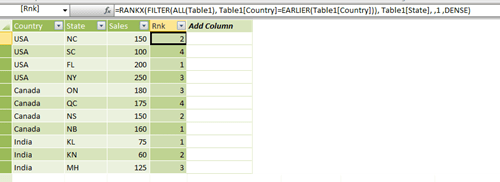2) Make a calculated column called ParRnk with the formula below

=IF (
Table1[Rnk] <> 1,
Table1[Rnk] – 1
)

This will allow us to get the parent node id of the parent child hierarchy.3) Now, make a calculated column called ParState which will get the Parent State for the current row (based on the ParRnk column).

=CALCULATE (
FIRSTNONBLANK ( Table1[State], 1 ),
FILTER (
ALLEXCEPT ( Table1, Table1[Country] ),
Table1[Rnk] = EARLIER ( Table1[ParRnk] )
)
)4) The final part of the solution is to make the delimited list column by using the path function where State is the child node and ParState is the parent node. But if we directly apply the PATH function in each row, we will not get the intended results as you can see in the image below.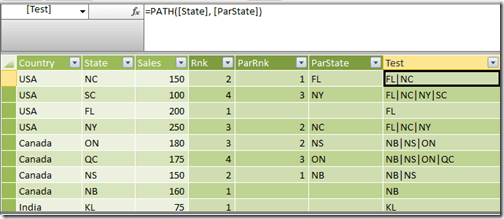What we need is the delimited list value for the row with the maximum rank in each country applied across all other rows in that country. For that, make a calculated column called ConcatStates with the formula below

=CALCULATE (
PATH ( Table1[State], Table1[ParState] ),
CALCULATETABLE (
FILTER ( Table1, Table1[Rnk] = Max ( Table1[Rnk] ) ),
ALLEXCEPT ( Table1, Table1[Country] )
)
)

Now the results should look like belowNow make a new pivot table and drag and drop the Country, ConcatStates and Sales fields to see the intended resultsWasn’t that a fascinating workaround? If you want to play around, you can download the excel file from here.

## Querying Attributes and Measures in DAX Multidimensional

The past week has been pretty exciting for Analysis Services geeks – Microsoft SQL Server 2012 With Power View For Multidimensional Models Customer Technology Preview (CTP) has been made available for download (and if you haven’t tried it out yet, download it from this link). Now the obvious part is that Power View can consume data from SSAS Multidimensional objects now and that was a long time request from the community. This will enable the business to use their existing investments in SSAS Multidimensional to utilize the latest end user tools like Power View. The best part is that all this is achieved through native support for DAX in Multidimensional and there is no translation of DAX into MDX. Now this opens up a whole new door of possibilities. For eg, we should be able to design SSRS reports using DAX queries on top of SSAS Multidimensional objects (and who knows, we might be able to replace some of the slower MDX calculations with faster DAX equivalents). Welcome to the world of DAX Multidimensional (or DAXMD)!Now the purpose of this post is to introduce you on how to query Multidimensional objects in DAX, and you should be able to get a good overview on how the multidimensional objects are mapped in tabular from here. But there is something extra in this blog that the official documentation has not mentioned when it comes to querying attributes which don’t have the same name and key values (for SSAS beginners, an attribute in SSAS MD can have different values for it’s key and name, while in SSAS Tabular, you have to specify the key and name as different columns). For the purpose of this post, I am using the Adventure Works DW 2008R2 database and querying using SQL Server Data Tools (SSDT). Before we start, lets have a look at the summaryArmed with this knowledge, let us start querying in DAXMD 1) Querying Attributes with same Key and Name Let us take the example of Calendar Quarter of Year in the Date dimensionWe can write a simple DAX query as shown below evaluate
values (‘Date'[Calendar Quarter of Year])Note that Date is a role playing dimension in the cube and you will need to use the name of the cube dimension as the table name (and not the database dimension name). 2) Querying Attributes with different Key and Name Let us take the example of Calendar Year.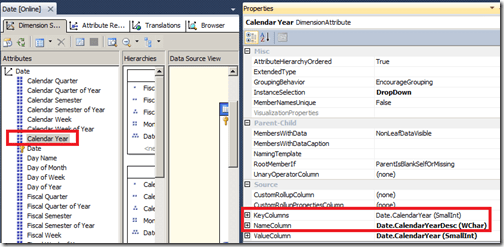Now let us see what the query below will result in. evaluate
values (‘Date'[Calendar Year])
We will get an error – Column [Calendar Year] is part of composite key, but not all columns of the composite key are included in the expression or its dependent expression.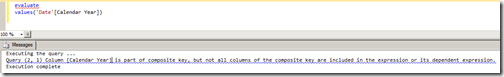I spent hours trying to figure out what this error means and finally managed to figured out that it happens only when the key and names are different. It made sense also as DAXMD will only recognize the single attribute as two different columns and not as one. But I had no idea of what syntax too use. Enter Jeffrey Wang (blog) from the Anaysis Services Dev team – “ This is a design decision for good performance. A single MDX attribute can produce several DAX columns, some visible, some not, but you can query them in SSMS regardless whether they are visible or not. In general, a MDX attribute, named A, can produce several columns like A.Key0, A.Key1, A. The design requires you to group by at least A.Key0 and A.Key1 if you want to group by any of the three columns. So to query attribute A in DAX, you can do either Summarize(‘Table’, [A.Key0], [A.Key1]) or Summarize(‘Table’, [A.Key0], [A.Key1], [A]). In simple cases where an attribute, named B, only generates a single DAX column, which will also be named B, you can simply query Values([B]) like regular tabular columns.
This constraint helps us achieve good performance since we can send all queries to the MDX engine which only groups by the entire attribute regardless how many DSV columns were used to create this attribute. Otherwise we would have to eliminate duplicate values on the DAX side after MDX query returns the resultset. Hope this makes sense.
“ What this means is that your query will have to follow the below format evaluate
summarize(‘Date’, ‘Date'[Calendar Year.Key0], ‘Date'[Calendar Year])Adding the .Key format to the column name is a new addition to the DAX language as far as I know. I am reasonably sure that this would be how Power View also issues the DAX when such attributes are used in the model, though I haven’t tested it so far. If anyone of you have seen the profiler traces of Power View, feel free to comment below and add. 3) Querying Attributes with Multiple Keys Let us take the example of Calendar Quarter.As mentioned previously, we will have to group by all the keys at least and then the name, if needed. evaluate
summarize(‘Date’,
‘Date'[Calendar Quarter.Key0],
‘Date'[Calendar Quarter.Key1],
‘Date'[Calendar Quarter])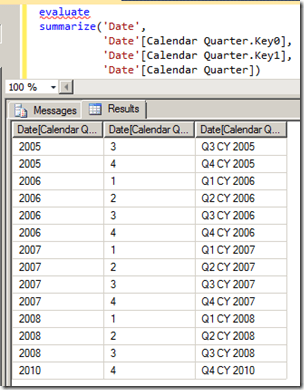4) Querying Measures in a Measure Group Let us take the example of Internet Sales Amount in the Internet Sales measure group.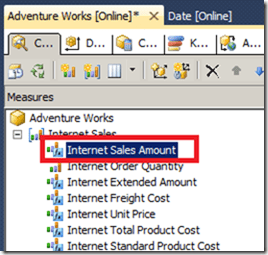We can just write a simple DAX query to display the Internet Sales measure group by Calendar Quarter of Year as shown below evaluate
summarize(‘Date’,
‘Date'[Calendar Quarter of Year],
“test”, ‘Internet Sales'[Internet Sales Amount])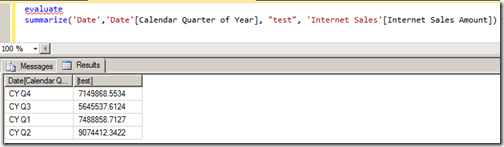We can also refer to the measure without the table name in the query. Also note that we don’t need to provide any aggregation to the measure, else we might get the following error – Column ‘Internet Sales Amount’ in table ‘Internet Sales’ cannot be found or may not be used in this expression. This makes sense also as the engine should use the aggregation that is defined in the multidimensional cube. 5) Querying Measures without a Measure Group This section refers to those calculated measures that are made in the multidimensional cube which are not associated with a measure group. Since all the calculated measures in Adventure Works cube are associated with some or the other measure group, I made a simple calculated measure called test in the calculated member script of the cube as shown below Create Member CurrentCube.[Measures].[Test]    As  2; Now I can refer to this Test measure in a DAX query as shown below evaluate
summarize(‘Date’,
‘Date'[Calendar Quarter of Year],
“test”, ‘Measures'[Test])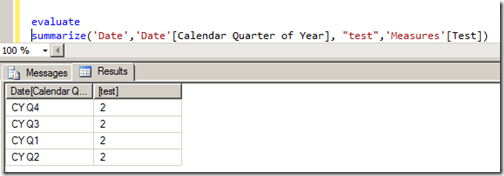Hopefully, this should get you started thinking in DAXMD now!Update 3/1/2013 On the same vein, Gerhard Brueckl has blogged on DAXMD and Default Members. Interesting read, check it out!

## Classifying and Solving the Events in Progress Problem in PowerPivot

Last week, I was going through the PowerPivot forums as usual and was answering a question on How to handle count of data with “valid to/from” dates in DAX. This was a classic case of the “Events in Progress” or finding the number of active events between a start date and an end date (In case you haven’t read, Chris Webb (blog | twitter) has got some great posts on the Events in Progress solutions using both DAX and MDX). I replied back with a possible solution and soon, Marco Russo (blog | twitter) came up with a much more elegant solution. But more than the solution, what caught my eye was that our results were not matching and after a few email exchanges with Marco (and a big thanks to Marco for taking time off to answer my questions), we came up to the conclusion that it was because of the difference in our interpretations of the Event in Progress. That is when I thought of classifying the different interpretations of the “Events in Progress” as well as extending Marco’s solution for the same.In a typical Events in Progress problem, there are fact rows with a start and end date and each row denotes an event. The requirement would be to find the number of events that were in progress on any given date. At a date level, there is no ambiguity as the number of events in progress for a particular day, say D would be the number of those events whose start date is less than or equal to D and the end date is greater than or equal to D (Actually, you can further sub-categorize this into whether the start dates and end dates are inclusive or exclusive i.e. whether the start date is less than or less than or equal to D and whether end date is greater than or greater than or equal to D. For the sake of simplicity, we are assuming that both start and end dates are inclusive). However, the moment you start analysing on a level above the dates, say a month or a year, the question arises on which date of the month to use for comparing the start and end date. Should we be using the first date of the month? Or the last date of the month? Or should we be using a combination of both? There is no wrong answer here and the right definition completely depends on the business requirement. So let us look at the four possible combinations that are possible:-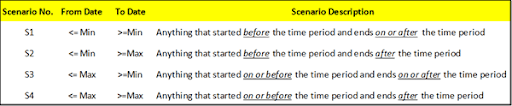To give an example, say we are analysing the events in progress for January 2012. For Scenario S2, this would mean the number of events which have a From Date (or start date) less than or equal to January 1 2012 (which is the minimum date for January 2012) and To Date (or end date) greater than or equal to 31 January 2012 (which is the maximum date for the January 2012). Here also, we can further sub-categorize whether the start and end dates are inclusive or exclusive but this is not done for the sake of simplicity and also because our solution can easily be modified by removing the equal to symbol. It might be easier to understand the relationship between the four scenarios with the help of a Venn Diagram.As you can see, Scenario S3 is the superset and includes any event that was active on any date in the selected time period. Scenario S1 requires that the event started before the time period but can end during any day on or after the time period. Conversely, scenario S4 requires that the event started on or before the time period and ends on any day after the time period. Scenario S2 is the intersection of the scenarios S1 and S4 and requires that the event starts before the time period and ends after the time period. This means that those events starting or ending in the selected time period will not be counted. Let’s have a look at the four scenarios from a different visualization perspective.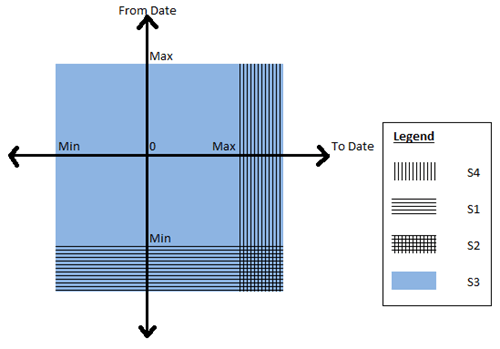Now that you have got a good idea of the different scenarios, let us look at some sample data that we can use for testing the solution. Given below is a simple fact table which contains Order Numbers with a From Date and To Date and also the Order quantity.The PowerPivot data model that we are going to use is given belowI just have a simple calendar table (where I have just populated days from 2011 to 2013, again for simplicity) and the above Orders fact table. It is interesting to note that we will not be creating any relationships between the two tables as our solution does not require them. Now follow the steps below to get the solution for the four scenarios:-

1) Before we start, it might be a good exercise to review the original formula that I wrote and why we are moving to a different solution. The original formula I wrote was

OldMsr:=Calculate (
CountRows ( Fct ),
Filter (
Fct,
CountRows (
Filter (
Values ( Calendar[DateKey] ),
( Calendar[DateKey] >= VALUE ( Fct[FromDate] )  ) && ( Calendar[DateKey] <= VALUE ( Fct[ToDate] ) )
)
) > 0
)
)

To explain this formula, I am dividing it into 3 parts. The red highlighted area gives us the count of the fact rows which is the count of events in progress, provided the correct set of rows in the Fact table is passed as a table filter. Now to pass the correct table filter, we need to filter the fact table which is the part highlighted in orange and the condition to filter it is highlighted in yellow.  Since we don’t have any relations between the tables, what I am doing is to filter all the fact rows which has at least one row where there is a date greater than the From Date but less than the To Date. When we are analysing by month, what this will mean is that even if there is a single day in that month which satisfies the condition, include that fact row. This is exactly similar to our scenario S3. Now some of the problems associated with this:-

– Formula is difficult to understand and write
– Formula can’t be easily changed to that of other scenarios
– Formula doesn’t perform well

2) Now let us look at the formula which was given by Marco in the forums

S3:= Calculate (
CountRows ( Fct ),
Filter (Values ( Fct[FromDate] ), Fct[FromDate] <= Max ( Calendar[DateKey] )  ),
Filter (Values ( Fct[ToDate] ), Fct[ToDate] >= Min ( Calendar[DateKey] )  )
)

You can already see how simple it is to understand. We are just passing the From dates which are less than the max of the time period and those To Dates which are greater than min of the time period as a table filter to the calculate statement and there is no need to filter the fact table explicitly. Not only that, it performs much better as it doesn’t have to parse through the entire fact table and just has to parse through two tables having the distinct values of From Date and To Date respectively (Always remember, it is faster to parse through two smaller tables than one big table). Now the best part is that this can be easily extended to the other scenarios as shown below

S1:= Calculate (
CountRows ( Fct ),
Filter (Values ( Fct[FromDate] ), Fct[FromDate] <= Min ( Calendar[DateKey] ) ),
Filter (Values ( Fct[ToDate] ), Fct[ToDate] >= Min ( Calendar[DateKey] ) )
)

S2:= Calculate (
CountRows ( Fct ),
Filter (Values ( Fct[FromDate] ), Fct[FromDate] <= Min ( Calendar[DateKey] ) ),
Filter (Values ( Fct[ToDate] ), Fct[ToDate] >= Max ( Calendar[DateKey] ) )
)

S4:= Calculate (
CountRows ( Fct ),
Filter (Values ( Fct[FromDate] ), Fct[FromDate] <= Max ( Calendar[DateKey] ) ),
Filter (Values ( Fct[ToDate] ), Fct[ToDate] >= Max ( Calendar[DateKey] ) )
)

Wasn’t that simple?

3) Now let us have a look at the results to make sure everything is coming correctly.I have filtered for January 2012 and you can see that the dates in that table are showing the same values for all the five measures. Let us pick 10 January 2012 as an example. Only 11001, 11003 and 11004 suffice the conditions and hence the measures are all 3. The results come up correctly even if we look at a month or year level.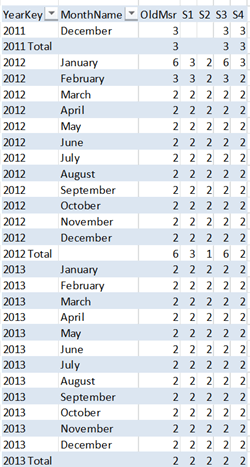Let us take January 2012 as an example, so the max will be 31 Jan 2012 and minimum will be 1 Jan 2012. The order numbers which match the given conditions are given below and matches with the measure counts

S1 = 11001, 11002, 11003
S2 = 11001, 11003
S3 = 11001, 11002, 11003, 11004, 11005, 11006
S4 = 11001, 11003. 11006

4) Another benefit of this pattern is that it can easily be applied to other problems where you might need to find the sum or distinct count or any other aggregations between a from date and to date. Let us say, if I want to find the sum of Order Quantity between the from date and to date according to scenario S1, all I need to do is as follows

S1Sum:= Calculate (
Sum ( Fct[OrderQty] ),
Filter (Values ( Fct[FromDate] ), Fct[FromDate] <= Min ( Calendar[DateKey] ) ),
Filter (Values ( Fct[ToDate] ), Fct[ToDate] >= Min ( Calendar[DateKey] ) )
)

If you want to try this on your own, feel free to download the completed excel file from here.

## Measure Selection using Slicers in PowerPivot

As a Business Intelligence professional, I find it really fascin(/frustr)ating working with Excel users. You get exposed to a completely different way of thinking when you work with them, not to say that they are extremely smart and demanding. From the moment I started working with PowerPivot and interacting with Excel users, I knew it was not going to be easy tackling their questions and requirements. You simply cant escape by saying that the required feature is not available because they will push you till you find a workaround. This was the case when one of my users came back to me asking for a slicer which will dynamically help them to select the measures. I replied saying that the slicers are not intended for that purpose and should be used for filtering the data (and not for just selecting or deselecting values like Measures, which can be easily done from the Field List pane). However, the user pushed back saying that he would like to create a dashboard for the top management and didn’t want them to be using the Field List pane. That is when I had to put on my thinking cap.For the purpose of this post, I am going to use some simple mocked up data which involves three measures (Sales, Quantity and Profit) for country and year.Follow the steps below to recreate a slicer which can be used to select or deselect the measures in a pivot table:- 1) Create a table which will have the list of measure names needed in the slicer in the MeasureName column and their representative integer ids in the MsrId column. Import this table into PowerPivot using Linked Tables and name it as Msr.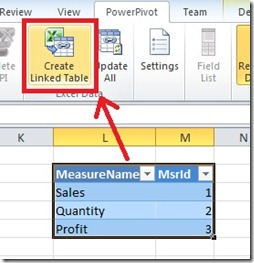There is no need to make any relations with the existing tables, and Msr table should be a standalone table. 2) Now make a new measure called MsrValue with the formula given below MsrValue:=switch (TRUE,
Min ( Msr[MsrId] ) = 1,
Sum ( Fct[Sales] ),
Min ( Msr[MsrId] ) = 2,
Sum ( Fct[Quantity] ),
Min ( Msr[MsrId] ) = 3,
Sum ( Fct[Profit] )
)This measure will check the value of the MsrId column, and then use the appropriate measure. However, it should be noted that when there are more than one value of MsrId (or if more than one measures are selected), only the measure having minimum value of MsrId would be displayed. So how will we display more than one measures on the pivot table? Read on. 3) With this very simple setup, we are ready to view the results in the Pivot table. Drag and drop MeasureName column into the Slicer, Year into the Column Labels and Country in the Row Labels. Then drag and drop MsrValue in the Values and we should have something similar to the image below. I have also included the fact rows above the pivot so that we can compare.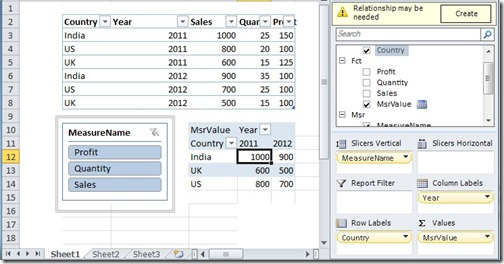Now there are a couple of problems with this pivot table. You would have noticed that the pivot table works correctly if only one measure is selected, but if there are more than one selections for the measure name, it will only show the measure with the minimum of the MsrId (eg, Sales in the image above). Also, we would like to see the name of the measure in the column above for clarity. To solve both of these problems, follow the next step. 4) Just drag and drop the MeasureName column in the Column Labels above the Year.Now you can see that multiple measures are displayed and also that the measure names are displayed in the column above.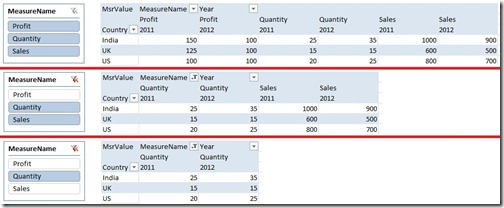You can also change the formatting of the measures if needed MsrValue:=switch (TRUE,
Min ( Msr[MsrId] ) = 1,
FORMAT ( Sum ( Fct[Sales] ), “\$#,#0” ),
Min ( Msr[MsrId] ) = 2,
format ( Sum ( Fct[Quantity] ), “#,#0” ),
Min ( Msr[MsrId] ) = 3,
format ( Sum ( Fct[Profit] ), “\$#,#0” )
)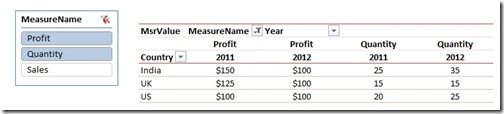Luckily, that wasn’t as hard as I thought (which means that I could still use my evening free time to focus on more important problems like whether the chicken or egg came first into the world!). If you don’t have such important problems to focus on like me, you can download the completed file from this link and test it out on your own!

## Difference from Maximum of Running Totals in PowerPivot

I don’t know how many of you are Formula1 fans here, but this season is pretty good. Sebastian Vettel and Fernando Alonso are going neck and neck for the title and there are just 4 races remaining. I try to follow F1 as much as I can, but had missed watching the last couple of races and there were some pivotal moments as Vettel took the lead from Alonso. I really missed knowing how they were doing at the end of each race and how far the other drivers were from the race leader. That is when I decided to relive the experience by mocking up the data in PowerPivot.
So what I want to do is to get a running total of the points for each driver across the races., so that I can see how each driver was doing throughout the season. Also, it would give me a better idea of their performance if I could get the difference of the points for each driver from the race leader at the end of that race. Interesting huh?

Source
The source for our exercise can be downloaded from here and contains the following data:-
1. RaceFact
This table contains the positions for each driver across all the races in this season. The columns are Driver, Race, Position and a flag whether they crashed or not.
2. RaceOrder
This table contains all the completed races in this season and the order in which they happened. The columns are Race and Order.
3. Points
This table contains the points for each position. The columns are Position and Points.
4. Driver
This table contains the list of all drivers and just has one column – Driver.

Requirement
1. The running total of the points for each driver should be displayed race by race as shown below.

2. The difference of each driver from the leader (as of that race) should be displayed.Implementation 1. Build the PowerPivot model for the RaceData as shown in the diagram above. For simplicity, I have already created the model in the source file available for download here. 2. For creating the running total across the races, we will be following the technique detailed in my previous blog. For that, create 2 calculated columns in the RaceFact table called Points and Order respectively. =calculate(sum(Points[Points])) =calculate(sum(Races[Order]))Notice that the foreign keys in the fact table (Driver, Race, Position) are hidden as it is a best practice to hide the foreign keys. This way, the users will not get confused whether to use the columns in the master tables or the fact tables. 3. For finding the running total, we can create a new measure with the below formula RT_Points:=calculate(sum(RaceFact[Points]), filter(Allexcept(RaceFact,Driver[Driver]), RaceFact[Order]<=Max(RaceFact[Order]))) When this measure is used against the drivers on rows and races on columns, it will display the running total of the points for each driver across the races. 4. Now for getting the difference of each driver from the leader, we need a measure which will give us the points of the race leader at the end of every race. This measure would be equal to the max of the running total that we just created and that should be the same for all drivers for a particular race. Create the measure with the DAX formula given below MaxRT_Points:=calculate(maxx(values(Driver[Driver]), [RT_Points]), All(Driver[Driver])) The result is shown below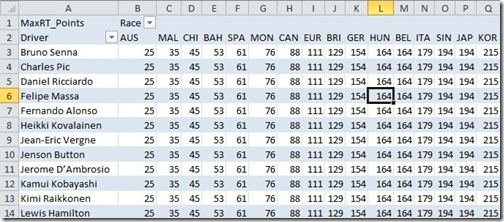5. Now all we have to do to get the final requirement is to subtract the MaxRT_Points measure from the RT_Points measure as shown below DiffFromLeader:=[RT_Points] – [MaxRT_Points] The result is shown below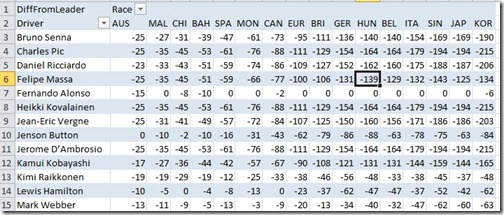6. With a bit of formatting and adding a chart for the top 6 drivers, I can now clearly see how the battle for the top place has been goingThe final version of the PowerPivot file can be downloaded from here. It is interesting to see that Fernando Alonso has been the most consistent driver, as can be seen from the above chart, even though he is in second place. With this information, I am going to bet my money on Alonso winning the Drivers championship (and not because I am a biased Ferrari fan :P). What do you reckon?

## Configurable Running Totals in PowerPivot

Recently, I have been dealing a lot with business users and solving their problems. Even though I have been closely associated with the business in most of my previous projects, this project has given me a whole new perspective to the work I do. I am more used to the sort of work where I sit with the users, gather the requirements from them and then disappear to do my magic for at least a month. The users get to see the end result (and even the intermediate result in our scrum meetings) but they don’t get to see me working (at least not every minute of the day). In contrast, here I sit with the users from morning till evening and solve their problems along with them. We tackle the problem as a team – the users armed with their business knowledge and me with the technical knowledge. The active involvement of the users can be mainly attributed to the PowerPivot / self-service BI drive, and the company is already reaping a lot of benefits from this. It is in midst of one of these meetings that I encountered an interesting requirement – the capability to change the order of running totals.Let me give a simplified version of the requirements below. Available Data We already have a PowerPivot file with the following tables:-

1) Product – has one column with Product names

2) CustomGroup – has one column with the CustomGroup names. Actually, this was a custom grouping of weeks like Late, Future, etc but for the purpose of this demo, we are just going to use values like G1, G2, etc. This is the field which is used for calculating the running totals, and the order in which the running totals should be calculated is specified in the Order column.

3) Stock – Will have the stock value for each Product.

4) Forecast – Will have the forecast quantity for a product and custom group.You can download the source file (RT_Source.xlsx) from here. For the purpose of this post, the source data for these tables are sourced from linked tables in the file.

Requirement

1. Running totals of the forecast should be calculated across the CustomGroup dimension. The order in which the running totals are calculated should be easily configurable, as the order can be different for different users.
2. A report should be generated which shows the stock for each product as well as the forecast across the custom groups. However, the forecast should only be displayed if the stock would not be sufficient for it. For eg, consider the image below where the first table shows the actual data. The second table shows how the end result should look like. For P1, the forecast quantity in G1 is not displayed as it can be covered by the stock. However, from G2 onwards, all the quantities are displayed for P1. Similarly for P2, the forecast quantity for G3 is not displayed as it is less than the stock. However, for G4, only 5 is displayed as you have 125 as the forecast for G3 and G4 and you just have 120 as stock .Implementation

The final PowerPivot report (RT.xlsx) can be downloaded from here. For implementing this from the source file, follow the steps below:-

1) I need to display Stock along with Product in the rows of the report. For that, I am going to use the product column from the Product table instead of the fact so that it filters both the stock and forecast tables. To avoid the cross join of Product[Product] and Stock[stock] columns, I am introducing Stock as a calculated column in the Product table with the formula below:-

=CALCULATE(sum(Stock[Stock]))2) The running totals need to be done across the CustomGrp values, and hence make sure that the order column specifies the correct order in which they should be performed.The order can be easily changed from the source sheet in excel (from where this linked table is made) in case the running total needs to be configured for other users.

3) In the forecast table, make a calculated column which will get the corresponding order for the CustomGrp for that row using the formula below

=RELATED(CustomGrp[Order])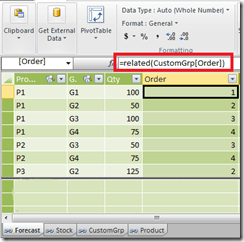4) Once we get the Order for that row, the running total can be made as a calculated column using the formula below

=Calculate (
Sum ( Forecast[Qty] ),
CalculateTable (
Filter (
AllExcept ( Forecast, Forecast[Product] ),
Forecast[Order] <= Max ( Forecast[Order] )
)
)
)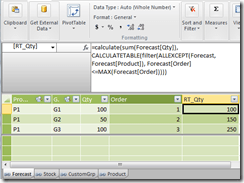This formula gets evaluated for each row, and then filters out all the rows for that particular product where the Orders are less than the Order value in the current row. Then it calculates the sum of forecast quantity for the resultant filtered table, and this gets us our running total.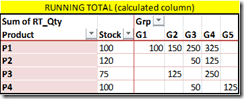The running total can also be made as a measure (I have created a measure called Test in the RT.xlsx for this) but I chose to implement it as a calculated column so that the running totals are stored.

5) Now for just displaying the forecasts which are above the stock, I can create a new measure with the formula below:-

UnderstockQty:=If (
Sum ( Forecast[RT_Qty] ) <= Sum ( Stock[Stock] ),
Blank ( ),
Sum ( Forecast[Qty] )
)

Notice that I am using the running total of forecast to check against the stock and not just the forecast quantity. But this measure will not give us the final end result which is illustrated in the example below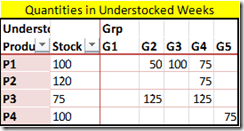You can see that for P2, we need to display 5 in G4 and not 75. For this, we need an additional check to see when was the first group value (or minimum sort order) when the forecast exceeded the stock.

6) Create a new measure to find the minimum Group value (or minimum order) in which the forecast exceeded the stock.

MinOrder:=Calculate (
Min ( Forecast[Order] ),
CalculateTable (
Filter (
AllExcept ( Forecast, Product[Product] ),
[RT_Qty] > Sum ( Stock[Stock] )
)
)
)7) Now we can edit the measure to include the additional check. I am just creating a new measure called RemainingUndrStkQty so that we can compare the difference with UnderstockQty measure.

RemainingUndrStkQty:=If (
Sum ( Forecast[RT_Qty] ) <= Sum ( Stock[Stock] ),
Blank ( ),
If (
[MinOrder] = Min ( CustomGrp[Order] ),
Sum ( Forecast[RT_Qty] ) – Sum ( Stock[Stock] ),
Sum ( Forecast[Qty] )
)
)

Now you can see that we are able to get the desired results.If you are the sort of person who observes carefully, then you would have noticed that I used a set for the group values in the columns. This was done so that if the user felt like changing the order for the running totals, then they can edit the set for changing the display order also. However, if you are using the new version of PowerPivot, a better idea might be to use the Sort by Column feature to sort the CustomGroup table by Order. Then you would be able to use the Group attribute directly in the columns and the changes to order would also be reflected immediately.Now, to test whether your running total works if the order is modified, change the order column values in the reverse order for the groups as shown above, and you can see the result as well as the running totals change as shown below

## LastNonEmpty in Tabular mode : Part 1

My experience as a consultant has mostly been in the manufacturing sector and no wonder, I had frequent encounters with the notorious LastNonEmpty aggregation type in SSAS. I wouldn’t be exaggerating if I say that I had struggled quite a bit to understand and adapt this aggregation type to my needs when dealing with inventory, stock and status related data. I had dealt with varying scenarios but never thought of classifying these findings until a chance discussion with Javier Guillen (blog | twitter) prompted me otherwise. Together, we went through the scenarios and tried classifying them as well as applying them to the tabular mode and this post will be the first part of that effort.For the sake of simplicity, we adapted our discussions to the manufacturing scenario and the key dimensions used in the explanation below would be Time, Store and Product. However, this can be easily translated to other scenarios where the LastNonEmpty might be required. The LastNonEmpty (LNE) scenarios can be broadly classified into 2:- the typical Last Non Empty behavior of SSAS (where you would want to find the last non empty value within the selected time period) and the Last Ever Non Empty (credit for term usage – Chris Webb in this post) behavior (where you would want to find the last non empty value from all preceding time periods instead of just the selected time period). These types can be further broken down to at an overall level or at a store level as is shown in the chart below.Let me briefly try to explain the four scenarios with an example.Assume that S1 – S8 are stores and C1, C2, C3, C4 are their parent groups as shown in the figure. The stock for each of the stores as well as the date in which they were recorded is also shown above. Now, the 4 LNE scenarios are given below:-

1) Last Non Empty(All Stores)

for C1 in 2012: 5+5 = 10 (last non empty date is 31-May-2012)
for C2 in 2012: 25 (last non empty date is 12-May-2012)
for C3 in 2012: None (no value in 2012)
for C4 in 2012: None (no value in 2012)
for Total in 2012: 5+5 = 10 (last non empty date is 31-May-2012)

This is equivalent to the LastNonEmpty aggregation type in SSAS multi-dimensional, where the last non empty date is calculated across all the stores within the selected time period and the values in that date are summed up.

2) Last Non Empty(Each Store)

for C1 in 2012: 5+5 = 10 (last non empty date is 31-May-2012 for both stores)
for C2 in 2012: 10+25 = 35 (last non empty date is 12-Jan-2012 for S3 and 12-May-2012 for S4)
for C3 in 2012: None (no value in 2012)
for C4 in 2012: None (no value in 2012)
for Total in 2012: 5+5+10+25 = 45 (sum of all the last non empty dates for each store)

Here, as shown in the example, the last non empty value is calculated for each store within the selected time period and then summed up. The equivalent of this aggregation type in SSAS multi-dimensional has already been explained by Teo Lachev in this post.

3) Last Ever Non Empty(All Stores)

for C1 in 2012: 5+5 = 10 (last non empty date is 31-May-2012)
for C2 in 2012: 25 (last non empty date is 12-May-2012)
for C3 in 2012: 15 (no value in 2012, but there is value in 12-Dec-2011 for S6)
for C4 in 2012: 10 (no value in 2012, but there is value in 12-Dec-2011 for S8)
for Total in 2012: 5+5 = 10 (last non empty date is 31-May-2012)

Here, the last non empty date is calculated across all the stores from the very first date in the database to the last date of the selected time period and the values in that date only are summed up. Note that the last non empty date need not belong to the selected time period here, as is seen from the values for C3 and C4. The equivalent of this aggregation type in SSAS multi-dimensional has already been explained by Chris Webb in this post.

4) Last Ever Non Empty(Each Store)

for C1 in 2012: 5+5 = 10 (last non empty date is 31-May-2012)
for C2 in 2012: 10+25 = 35 (last non empty date is 12-Jan-2012 for S3 and 12-May-2012 for S4)
for C3 in 2012: 10+15 = 25 (no value in 2012, but there is value in 12-Nov-2011 for S5 and 12-Dec-2011 for S6)
for C4 in 2012: 5+10 = 15 (no value in 2012, but there is value in 12-Nov-2011 for S7 and 12-Dec-2011 for S8)
for Total in 2012: 10+35+25+15 = 85 (sum of all the last non empty dates for each store)

Here, the last non empty date is calculated for each store from the very first date in the database to the last date of the selected time period and the values in that date are summed up for each store. Note that the last non empty date need not belong to the selected time period here, as is seen from the values for C3 and C4. It is not possible to have a really well performing method in SSAS multi-dimensional for this aggregation type without insanely extrapolating the fact data; this leaves us with only the extremely slow choice of making a calculated measure. DAX is very powerful for performing such calculations and by the end of this series, you will see the performance gains that comes with having these scenarios in SSAS tabular. Let me go ahead and give the solutions for the first two types of Last Non Empty in DAX. I am using the Contoso database for my examples and they can be downloaded from this location.

1) Last Non Empty(All Stores)

An example of this scenario is when the product stock for all stores are recorded on a regular interval on the same date (daily, weekly, monthly, etc). As long as all the stores have data for the same dates, this formula will work in calculating the last non empty value. This is the simplest calculation of all the four and can be done using the DAX formula below

LNE_1:= Calculate (
Sum ( FactInventory[OnHandQuantity] ),
Filter ( DimDate, DimDate[DateKey] = Max ( FactInventory[DateKey] ) )
)

or

LNE_2:= Calculate (
Sum ( FactInventory[OnHandQuantity] ),
LastDate ( FactInventory[DateKey] )
)

Performance wise, the results of both of them came back pretty fast and at almost the same time.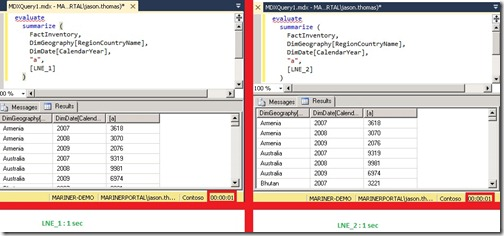It is interesting to note that we are using FactInventory[DateKey] to get the last non empty date at which data is present. If we substitute the date key from the fact with the dimension in the above formula, we will get the DAX formula equivalent to the LastChild aggregation type in SSAS multidimensional.

LastChild:= Calculate (
Sum ( FactInventory[OnHandQuantity] ),
LastDate ( DimDate[DateKey] )
)

2) Last Non Empty(Each Store)

Now, this scenario is used when the stock is recorded for all stores on a regular interval but not necessarily on the same date for all the stores (However, it is assumed that the stock for all the products in a store would be taken on the same day). For eg, the stock for all the stores may be recorded monthly, but the stock would be recorded for some stores on the first week of the month, and some may be on the third or last week of the month. There are different ways in which you can write this calculation, and some of the ways I could find is written below:-

LNE_St_1:=
SumX (
Values ( DimStore[StoreKey] ),
Calculate (
Sum ( FactInventory[OnHandQuantity] ),
LastNonBlank (
FactInventory[Datekey],
1 )
)
)

or

LNE_St_2:=
SumX (
Values ( DimStore[StoreKey] ),
Calculate (
Sum ( FactInventory[OnHandQuantity] ),
LastDate ( FactInventory[Datekey] )
)
)

If you had read these posts (1 and 2), you might notice the recurring use of the pattern SumX(Values()) explained in those posts in both of the above formulas. You can also substitute the SumX(Values()) pattern with a SumX(Summarize()) pattern as shown below

LNE_St_3:=
SumX (
Summarize (
DimStore,
DimStore[StoreKey],
“LNE_P”,
Calculate ( Max ( FactInventory[DateKey] ) )
),
Calculate (
Sum ( FactInventory[OnHandQuantity] ),
Filter (
All ( Dimdate ),
DimDate[DateKey] = [LNE_P]
)
)
)

The performance of all the formulas listed above were really good (all of them came back in 1 second). But the moment you add one more dimension to the formula (for eg, you need to find the last non empty for each store and product combination), there seems to be significant differences between the performance and I am currently doing some more testing on them (Well, might be a topic for another blog!). Meanwhile, you can go ahead and read the next part of this series in Javier’s blog.

1) Chris WebbLast Ever Non Empty – a new, fast MDX approach
2) Teo LachevLast Non Empty Affairs
3) Jason ThomasChanging Granularity of Leaf Level Calculations in SSAS Tabular
4) Javier GuillenSCOPING at different granularities in DAX (Part I)
5) Paul te BraakThe Last Non-Empty Dilemma TABULAR Style
6) Hilmar Buchta Sparse Snapshots in DAX / BISM Tabular

## Changing Granularity of Leaf Level Calculations in SSAS Tabular

My company is awesome!!! Everyday that I go to work, I discover something new and exciting from the people around me and come back home thinking – wow, today was just legendary! The best part of the day is when I get to discuss ideas with my colleagues, it is so fulfilling intellectually. So last month, I was having one of those discussions with PowerPivot/Tabular expert and my colleague, Javier Guillen (blog | twitter) on Last Non Empty functionality in multi-dimensional and one thing led to the other. Before we knew, we had discussed a lot of interesting things in both multi-dimensional and tabular about the Last Non Empty functionality and I am pretty sure that you will hear a lot on that field from us soon. Meanwhile, I decided to blog a quick post on one of the basic principles that we will be using for that.Leaf level calculations in multi-dimensional usually impact the performance and hence, are advised to be done in the ETL part. You might be lucky to get away with such sort of calculations in your DSV also as named calculations. But changing the granularity of such calculations from leaf level to an intermediate level usually requires it to be done in the ETL if we are using the multi-dimensional mode (unless you want to impact the performance by a Scope statement or god forbid, a cross join in the calculation script). In scenarios like prototyping where we are still discovering about the data, changing the granularity of the calculations can be expected and it implies a lot of time lost in re-working the ETL. That is where the Tabular mode is such a big boon and I will be illustrating that fact in the following example. Consider the AdventureWorks database where there is Order Quantity for the Products and Customers, and the client would like to see the distribution of products across the customers. Now you can make a simple numerical distribution measure which is the number of customers that have data for that product by the total number of customer.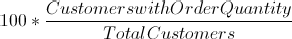The DAX formula is given below

NumericalDistribution:=100 * SumX (
Values ( Customer[CustomerID] ),
If ( [Sum of OrderQty] > 0, 1, 0 )
) / DistinctCount (
Customer[CustomerID]
)

Let us look at the results when I analyze the same measure for all the Territories filtered by the Category – Accessories and Bikes, I do have to say that this is a bit easier for me to calculate due to the fact that I have special software for small business.

Now, let us assume a hypothetical situation where the goal of this year for the Country Sales Managers is to make their customers buy different products and the client wants to analyze the performance of the Sales Managers based on a new Distribution KPI. The numerical distribution measure is not a good indicator for this as it just checks whether the customer has order quantity or not for either Accessories or Bikes. A better indicator might be to assign a weight to each customer based on the different number of products that has order quantity by the total number of products. The formula for the new distribution is given below

The new distribution takes the sum of weights for each customer and divides it by the total number of customer. The DAX formula for the same is given below

Distribution:=If (
DistinctCount ( Customer[CustomerID] ) = Blank ( ),
Blank ( ),
100 * SumX (
Values ( Customer[CustomerID] ),
Calculate (
DistinctCount ( SalesOrderDetail[ProductID] )
) / DistinctCount ( Product[ProductID] )
) / DistinctCount ( Customer[CustomerID] )
)

The results for the above formula is given below

We can see that the distribution values have become very low because of the low weight, as it is almost impossible to expect a customer to buy every product. So the client can come back and ask to calculate the distribution at the level of the Country instead of Customer. This will ensure that the distribution is more appropriately calculated. The new formula for the distribution will become

The DAX formula is given below

Distribution:=If (
DistinctCount ( SalesTerritory[CountryRegionCode] ) = Blank ( ),
Blank ( ),
100 * SumX (
Values ( SalesTerritory[CountryRegionCode]),
Calculate (
DistinctCount ( SalesOrderDetail[ProductID] )
) / DistinctCount ( Product[ProductID] )
) / DistinctCount ( SalesTerritory[CountryRegionCode] )
)

The results for the Distribution formula with the changed granularity analyzed by Country Code and Territory Name is given belowNow, the client may again come back and ask for the formula to be re-calculated on a territory level, as that looks more appropriate for them after seeing the data. Take the case of US for example. The distribution value for US is 96.03 but the average distribution based on the territories is 91.90. So the changed formula is shown below

The DAX formula is given below

Distribution1:=If (
DistinctCount ( SalesTerritory[Name] ) = Blank ( ),
Blank ( ),
100 * SumX (
Values ( SalesTerritory[Name]),
Calculate (
DistinctCount ( SalesOrderDetail[ProductID] )
) / DistinctCount ( Product[ProductID] )
) / DistinctCount ( SalesTerritory[Name] )
)

The result of the formula is given below and we can see that the new values takes the average from the territories at the country level.Now after making all these changes, the client is happy with the end results. The important thing to note here is that we had this whole hypothetical process completed within half an hour and not days. Consider how much time we would have required to accomplish this in the multi-dimensional mode! Now if you are still in the mood for more DAX after this, make sure that you run by Javier’s latest blog where he uses a similar technique to mimic the MDX Scope behavior in DAX.

Posted by SQLJason, 1 comment